# Miscellaneous FAQs

## My Names window looks different to the screenshots in the training materials and I can't find all the buttons mentioned in the instructions

Example question: I'm using MLwiN v2.18 and working through your LEMMA online training materials. What I see in the Names window looks different to the screenshot given. When the instructions ask me to press Categories I don't know what to do because I can't see this button, and when they ask me to press Copy I don't know what to do because there are two Copy buttons.

The training materials and manuals available on our main website and as part of our LEMMA online learning materials were all created using earlier versions of MLwiN than v2.18. In MLwiN v2.18 there have been some changes to the Names window. ( Further details…)

The changes affect the appearance of the Names window; some buttons have changed their location, and some buttons have also changed their name. There are also some new features available in the Names window; there is no loss of features compared to earlier versions (every facility offered by the Names window in v2.10 to v2.17 is also available in v2.18).

Since the training materials use screenshots and instructions based on the earlier versions, users should be aware that:

• screenshots of the Names window will look slightly different to what they will see when using v2.18
• instructions may ask them to press a button which does not exist under that name in v2.18 (for example Categories which is now View under Categories), or refer to a button name that appears in two different places (for example Copy which now appears both under Data and Categories)
• when working with category labels instructions may take users through a process that is slightly more laborious than the easiest way to achieve the same effect in v2.18

We do intend to update many of our materials, in particular the manuals and the LEMMA online learning materials. However this is a fairly large undertaking and it is anticipated that this will not happen for several months. In the meantime we ask users to be aware of these small differences between the latest version of MLwiN and the training materials, and apologise for any confusion resulting from this.

## Is it possible to fit splines in MLwiN?

Yes, it is possible to fit splines in MLwiN, but there is no special facility for doing this: it has to be done manually.

1. Create an indicator variable for each interval you want to fit a different polynomial to. In other words, if the cut points for your spline are 2, 7, 10, and expvar is your explanatory variable, and c20 onwards are all free columns then type in the Command interface:

calc c20 = 'expvar' < 2
calc c21 = ('expvar' >=2) & ('expvar' < 7)
calc c22 = ('expvar' >= 7) & ('expvar' < 10)
calc c23 = 'expvar' >= 10

name c20 'int1'
name c21 'int2'
name c22 'int3'
name c23 'int4'

(Or you can use the Recode by range window with a new destination variable each time)
2. Create a new explanatory variable for each interval by multiplying 'expvar' by the indicator variable

calc c24 = 'expvar' * 'int1'
calc c25 = 'expvar' * 'int2'
calc c26 = 'expvar' * 'int3'
calc c27 = 'expvar' * 'int4'

name c24 'expvar1'
name c25 'expvar2'
name c26 'expvar3'
name c27 'expvar4'
3. Add each explanatory variable as a polynomial (first adding in the indicator variable for that interval to give each polynomial a different intercept)
• Click Add Term at the bottom of the Equations window
• Select int1 and click Done
• Click Add Term again, select expvar1, tick the polynomial box and select the appropriate poly degree from the drop down box. Click Done
• Repeat for the other 3 intervals
4. Set up constraints: the spline needs adjacent polynomials to have the same value and the same derivatives (up to the (n-1)th, where n is the degree of the polynomials) at the point where they meet. If there are random effects on any of the terms in the spline, we also need to make sure that the variance at each level is the same for adjacent polynomials at the point where they meet. For details of how to specify constraints on the parameters see the FCON and RCON commands in the Command manual, or in the Help see Introduction to MLwiN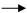MLwiN InterfaceMenu itemsModel MenuConstrained parameters.

For example, if we have a cubic for each polynomial and have added the terms in the order described above so that the Equations window shows

response =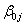int1ij +expvar1^1ij +expvar1^2ij +expvar1^3ij +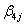int2ij +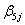expvar2^1ij +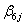expvar2^2ij +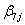expvar2^3ij +…+ eij cons,

and we have a level 2 random effect on each intercept and linear term (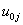int1ij,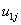expvar1^1ij,int2ij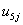expvar2^1ij,…),  then to deal with the first join, between interval 1 and interval 2, we need 4 constraints:

These polynomials meet at expvar1 = expvar2 = 2. The first constraint is that the value of the polynomials should be the same at this point, so we need+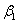× 2 +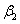× 22 +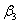× 23  =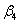+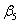× 2 +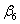× 22 +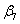× 23
Our constraint will be+ 2+ 4+ 8-- 2- 4- 8= 0.
The second constraint is that the first derivatives of these polynomials are equal at this point, so we need+ 2× 2 + 3× 22  =+ 2× 2 + 3× 22
so our constraint will be+ 4+ 12-- 4- 12= 0
Similarly our third constraint, that the second derivatives of these polynomials are equal at 2, will be 2+ 12- 2- 12= 0.
Our fourth constraint is that the level 2 variance due to the random effects on the first polynomial and the level 2 variance due to the random effects on the second polynomial should be equal at expvar = 2. In other words,× 1 +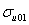× 1 × 2 +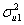× 22 =× 1 +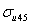× 1 × 2 +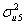× 22
Our constraint will be+ 2+ 4-- 2- 4= 0.

We will have two further similar sets of four constraints to make sure the value, derivatives and level 2 variance are equal at the other cut points.

## My level 2 variables are being treated as level 1 variables

Example question: I have some level 2 variables (i.e. variables which have the same value for all individuals in the same level 2 unit) which I put in my model as explanatory variables. The Equations window shows some of these with an ij subscript, not a j subscript, indicating that MLwiN thinks these are level 1 variables. How do I tell MLwiN they are level 2 variables?

MLwiN works out automatically whether a variable is a level 2 variable. It does this by checking whether there is any level 2 unit where not all individuals have the same value of the variable. If there is (at least 1) level 2 unit like this, then it concludes that the variable is a level 1 variable. If there are no level 2 units like this, so that in all level 2 units all individuals have the same value of the variable, then it concludes that the variable is a level 2 variable.

If MLwiN gives one of your level 2 variables an ij subscript in the Equations window, showing that it thinks this variable is a level 1 variable, then you should check for mistakes in your data: a level 2 unit where not all individuals have the same value of the variable. You can do this by inspecting your data, of course, but a quicker and less error-prone method is as follows (to check a level 2 variable called popden):

From the Data Manipulations menu, select Multilevel data manipulations. In the window that appears, under Operation select Minimum and under On blocks defined by select the column which contains your level 2 identifiers. Under Input columns select popden and under Output columns select any free column (say c70). Select Add to action list and Execute. Now from under Operation select Maximum and select another free column (say c71), leave everything else the same and click Add to action list and Execute again. Now c70 contains the smallest value of popden for each level 2 unit and c71 contains the largest value of popden for each level 2 unit. If popden is supposed to be a level 2 variable and if there are no mistakes in the data then c70 should be identical to c71. We check this by typing in the Command interface window (available from the Data Manipulation menu) 'calc c72=c71-c70' -that is we are calculating the difference between c70 and c71 which we expect to be 0 for every row. We can now check whether the data are correctly entered by looking in the Names window. If c72 is 0 for every row then in Names window in the row for c72 we will see 0 under min and 0 under max. If we do not this tells us that in at least one case, c70 and c71 were not identical, so the minimum and maximum values of popden were not equal for each level 2 unit- i.e. for some level 2 unit different level 1 units had different values of popden. In order to find out which level 2 unit (and whether this is the case for more than one level 2 unit), we generate a column that will keep track of which row things are on. We do this by selecting Generate vector from the Data Manipulation menu, selecting Sequence under Type of vector, selecting a free column (e.g. c73) next to Output column, typing 1 next to Start number, the length of the dataset next to End number, and 1 next to Step value, and selecting Generate. Now from the Data Manipulation menu select Select or omit cases, under Condition to select or omit on type c72 != 0, under Input columns select c72 and c73 and under Output columns select two free columns (e.g. c74 and c75), and click Add to action list and Execute. We have now created two new columns which have kept only information from rows where c72 was not 0; c74 contains the value of c72 for those rows and c75 contains the value of c73 for those rows (and will tell us which row of the original dataset the values came from). If we look at c74 and c75 in the Data window we can see the nonzero values of c72 and which row of the original dataset each comes from. Now if we look at these rows in our original dataset (looking at the column with the level 2 IDs and popden) we should be able to see the discrepancy, and edit the data appropriately.

Note that in some cases we may find that we got a nonzero value simply because the value of popden was missing (then the corresponding value in c74 will be MISSING). If the value of popden is missing for all individuals in a level 2 unit, then we will find entries for these individuals in c74 and c75, but this will not stop MLwiN treating popden as a level 2 variable. If, on the other hand, the value of popden is missing for only some individuals in a level 2 unit, then MLwiN will treat popden as a level 1 variable.

## MLwiN can no longer PRINT windows

Example question: I upgraded my MLwiN to version 2.20 and no longer can PRINT windows! When I select FILE, the PRINT option does not appear. Has anyone else encountered this? I had no errors appear when I installed the upgrade. I was using an earlier version earlier this morning and PRINT did appear (and I used it). Please let me know what to do.

The window capturing functionality has been moved to a (so far undocumented) command. This will allow window capture to be performed as part of a macro, as well as adding the ability to capture window images directly to bitmap files. Unfortunately the new command means that we are no longer able to send a window image directly to the printer. You can however get the same effect (with possibly more control over the size on paper/etc) by capturing the window image with the "EditCopy" menu option and then pasting the image into another application, such as a word processor, and printing from there.

Note: some of the documents on this page are in PDF format. In order to view a PDF you will need Adobe Acrobat Reader